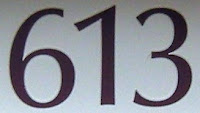## Friday, October 14, 2011

### 613

613 is a prime number.

613 is the smallest prime such that bringing the first digit to the back produces a triangular number (136) and repeating this operation produces a square (361).

613 is the smallest number n such that the numbers of divisors of n, n + 1, n + 2, and n + 3 are k, 2k, 4k, and 8k for k = 2.

613 has a representation as a sum of two squares: 613 = 172 + 182.

613 is the hypotenuse of a primitive Pythagorean triple: 6132 = 352 + 6122.The 613 commandments (613 Mitzvot) is a numbering of the statements and principles of law, ethics, and spiritual practice contained in the Torah (the Five Books of Moses).